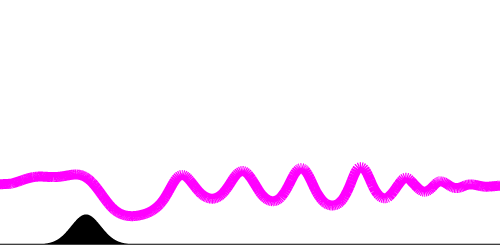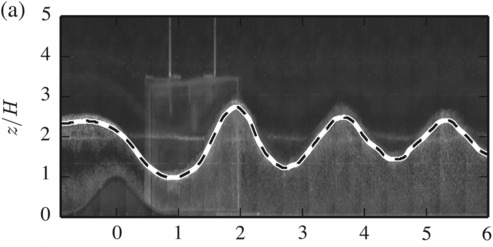# sandbox/Antoonvh/lee.cMountain waves can induce lenticular clouds. Photo courtesy of Jacob Kollegger, hosted via skybrary.

# Lee waves

A stably stratified flow over a mountain can cause a particular wave pattern to arrise. These periodic motions are known in literature as “Lee waves”, after their localization in space; i.e down wind from the geometric feature. Subsequently, the Lee-side of a mountain is named after J.T. Lee, who studied waves in stratified fluids induced by flows over mountainous geometries.

## The phyiscal Setup

The aim is to create a 2D analogue of the laboratory set-up of Stiperski et al. (2017). The setup consists of flow over a Gaussian mountain with height H and width \sigma by a fluid with a reference buoyancy b_{r}. The fluid is characterized by a two-layer buoyancy (b) structure that is described by a neutrally stratified layer near the surface of height y_h and a less dense fluid with a jump in the associated buoyancy over a depth d at the interface (b_2 = b_r + b'), with a constant stratification aloft. The statification strength is expressed by the Brunt-Vaisiala frequency (N). Introducing,

\displaystyle \Pi_1 = \frac{H}{\sigma} = 0.57 \displaystyle \Pi_2 = \frac{H}{d}=20, \displaystyle \Pi_3 = \frac{HN}{U}=0.5, \displaystyle \Pi_4 = \frac{H}{y_h}=0.5, \displaystyle Fr = \frac{U}{\sqrt{b'h_y}}= 0.67

Corresponding to experiment number 203 of Stiperski et al. (2017).

double Pi1 = 0.57;
double Pi2 = 20;
double Pi3 = 0.5;
double Pi4 = 0.5;
double Fr  = 0.67;

In the original lab experiment, the flow over the mountain adjusts near the mountain’s surface to the moving obstable due to the fluid’s viscosity (\nu), within a so-called boundary layer. This warrants the introduction of a Reynolds number. We choose,

\displaystyle Re = \frac{UH}{\nu}= 4000.

This value is large enough that it would allow a 3D turbulent wake to develop in the experiment. This is obviously not captured in our 2D analogue set-up.

double Re = 4000;

Note also that in our numerical experiment we use stress-free condition at the bottom surface.

## The Numerical Setup

The mountain is embedded into fluid domain that is governed by the Navier-Stokes equations. Furthermore, we like to view our computations.

#include "embed.h"
#include "navier-stokes/centered.h"
#include "tracer.h"
#include "view.h"

A buoyancy tracer field is declared:

scalar b[];
scalar * tracers = {b};
b[top] = neumann (sq(Nb));

we use a normalized value for the mountain height and the fluids’ speed with respect to the mountain.

#define MOUNTAIN (exp(-(sq(x + L0/4.)/sq(2./Pi1))) - y)
#define STRATIFICATION  ((bacc*(1. + (tanh((y - yh)/d)/2.))) + ((y > yh)*(y - yh)*sq(Nb)))
double Nb, yh, bacc, d, eb;
int maxlevel = 10;
face vector av[];
face vector muc[];
scalar f[];

For the domain, a 100H \times 100H box is used that is discretized with a maximum of 1024 cells in each spatial direction. The simulation is run for 80 units of time (t_{end} = 80 \times H/U) Furthermore, the values for stratification strength, the height of the two-layer interface and the density jump according to the chosen values for the dimensionless groups are set. To limit the most prominent issues with inconsistent in/out flow boundary condtions, periodicity is prescribed in the lateral direction.

int main() {
periodic (left);
Nb = Pi3;
yh = 1./Pi4;
bacc = 1./(yh*sq(Fr));
d = 1./Pi2;
L0 = 100.;
X0 = -L0/2.;
eb = 0.025*bacc;
a = av;
mu = muc;
init_grid (1 << 8);
run();
}

event properties (i++) {
foreach_face()
muc.x[] = fm.x[]/Re;
}

## Initialization

The set-up is initalized with a left-to-right oriented velocity and the buouancy is defined by the STRATIFICATION macro. Since we do not define a consistent modified pressure, we start with a small tolerance on the Poisson problem’s residuals. Furthermore, the mountain is embedded into the domain via the MOUNTAIN macro.

event init (t = 0) {
refine (fabs(MOUNTAIN) < 0.2 && level < maxlevel - 1);
refine (fabs(MOUNTAIN) < 0.1 && level < maxlevel);
refine (y < yh + 0.2 && y > yh - 0.2 && level < maxlevel);
vertex scalar phi[];
foreach_vertex()
phi[] = -MOUNTAIN;
boundary ({phi});
fractions (phi, cs, fs);
foreach() {
u.x[] = cs[];
b[] = STRATIFICATION*cs[];
}
TOLERANCE = 1e-5;
}

event back_to_normal (i = 10)
TOLERANCE = 1e-3;

## The effects of Gravity

Using the buoyancy formulation is not only nice for the argument of generality. Also, it provides an easy-enough framework to implement the effects of gravity.

event acceleration (i++) {
foreach_face(y)
av.y[] = fm.y[]*(b[] + b[0, -1])/2.;

An inlet profile is mimicked by forcing the velocities and buoyancy field at the left-hand-side of the domain.

  foreach() {
if ( x < X0 + L0/8.) {
u.x[] += 0.5*dt*(1 - u.x[]);
b[] += 0.5*dt*(STRATIFICATION - b[]);
}
}
}

Each timestep, the grid is adapted with respect to the wavelet-based estimated discretization error for the representation of the buoyancy field, the velocity components fields and the representation of the embedded boundary.

event adapt (i++)
(double[]){0.05, 0.05, eb, 0.001}, maxlevel);

## Output

The foremost important output consists of a movie displaying the obstacle and the vertical gradient in the buoyancy field (dbdz,\frac{\partial b}{\partial y}). We also visualize the used adaptive grid strucure.

event bviewer (t += 0.1) {
double disph = L0/8.;
scalar dbdz[], m[];
foreach() {
if (y > disph)
dbdz[] = nodata;
else
dbdz[] = ((b[0,1] - b[0,-1])/(2*Delta));
}
clear();
view (fov = 6, tx = (-0.6/L0), ty = -0.15,
width= 1000, height = 400,  sx = 1.);
draw_vof ("cs", "fs", filled = -1, fc = {1, 1, 1});
squares ("dbdz", min = sq(Nb)/2., max = bacc/(4*d));
begin_translate (y = 1.1*disph);
cells();
end_translate ();
save ("lee.mp4");
}

We can view the movie:

Buoyancy gradient and the grid structure in the co-moving frame.

We also output an image depicting the interfacial wave by plotting an iso-line of the b=b'. To allow for comparison, the x-direction is scaled with the mountain’s so-called half-width (L).

 event image (t = 80) {
scalar ff[];
vertex scalar phi[];
foreach_vertex()
phi[] = interpolate (b, x, y) - bacc;
fractions (phi, ff);
clear();
view (fov = 1.6, tx = (-0.5/L0), ty = -0.04,
width= 500, height = 250,  sx = 1./5.);
draw_vof ("cs", "fs", filled = -1, lc = {0, 0, 0});
draw_vof ("ff", lc = {1, 0, 1}, lw = 10);
box();
save ("leeimage.png");
}

We can compare the results from our simulation against those obtained by Stiperski et al. (2017), as presented in J Sachsperger (2017)Our resultSnapshot taken during experiment nr. 203, as presented in J Sachsperger (2017) (CC 4.0 licence)

The results look OK.

To check if (or by how much) the simulation benefits from grid adaptivity, we count the used number of grid cells and compare it against those of a square and equidistant-resolution grid.

event grid_monitor (i += 10) {
static FILE * fp = fopen ("cells", "w");
int n = grid->n;
fprintf (fp, "%g\t%d\t%d\t%g\n",
t, i, n, (double)(1<<(depth()*dimension))/(double)(n));
fflush (fp);
}

We can view the results,

set yr [0:120]
set xlabel 'Solver iteration'
set ylabel 'reduction factor'
set key off
plot 'cells' u 2:4 w l lw 3(script)

A healthy reduction factor is obtained. It would be interesting to reformulate the problem with the reduced gravity approach for the inversion layer.

Stiperski, I., Serafin, S., Paci, A., Ágústsson, H., Belleudy, A., Calmer, R., … & Grubišić, V. (2017). Water tank experiments on stratified flow over double mountain-shaped obstacles at high-reynolds number. Atmosphere, 8(1), 13.

Sachsperger, J., Serafin, S., Grubišić, V., Stiperski, I., & Paci, A. (2017). The amplitude of lee waves on the boundary‐layer inversion. Quarterly Journal of the Royal Meteorological Society, 143(702), 27-36.